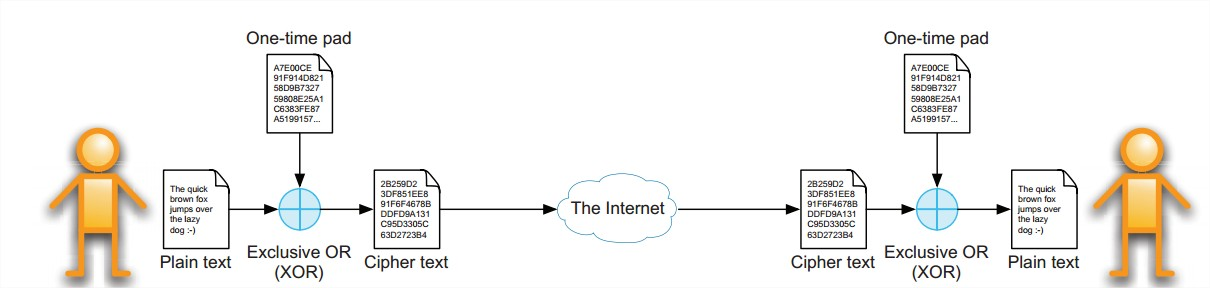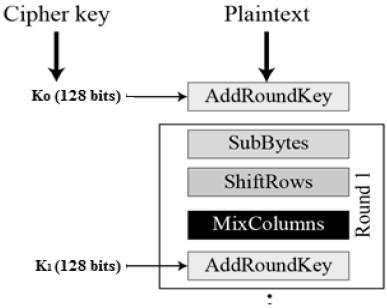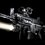# Mathematics Behind The Cryptography And It's Basic Principles--2

Symmetric cryptography :

Symmetric cryptography is the most widely used form of cryptography. It can be used to secure communication by two or more parties and relies on a secret that is shared between the parties. Symmetric key algorithms are a fast way to securely encrypt data using a shared secret. The two most commonly used algorithms to date are Triple DES and AES.

Symmetric key algorithms exist in two variants:

Block ciphers– these operate on groups of bits called blocks; a block cipher typically has a fixed block size that is a multiple of 8 bits (common block sizes are 64 bits and 128 bits)

Stream ciphers– These operate on single bits of data.

A symmetric key algorithm is called ‘symmetric because the same key is used in both the encryption as well as the decryption operation.Given a good symmetric key algorithm the security depends on the shared secret key remaining secret. Once the key has been disclosed, any eavesdropper can decrypt the message traffic between the communicating parties.The security of block ciphers depends on the ability of the algorithm to create an output that is indistinguishable from truly random data and on the randomness of the key.

The big disadvantage of stream ciphers is that their security is heavily dependent on the quality of the pseudo random number generator that generates the key stream. A number of stream ciphers (including – for instance – RC4, which was used for SSL and Wi-Fi WEP encryption) have been broken because of this.

There are Many Algorithms Of Symmetric cryptography . Some Of Them Are Explained Bellow :

The one-time pad is the only perfectly concealing cryptographic algorithm that completely hides the plain text and offers no chance of recovering the plain text by brute force without trying all possible pads of the same length as the cipher text which is usually completely infeasible. The mechanism is based on using a truly random set of data that is exactly the same length as the plain text that has to be encrypted.

The random data used to encrypt may only be used once (hence the ‘one-time’ in the name). Traditionally this random information was stored on pieces of paper (hence the word ‘pad’ in the name).The one-time pad data is often used to encrypt the plain text using a simple binary exclusive-or (XOR)operation; this yields the cipher text. The advantage of using the exclusive-or operation is that it is a native instruction on almost all computer systems and operates very efficiently.The receiving party uses the same one-time pad data to perform the XOR operation on the cipher text, which will yield the plain text.The workings of one-time pads are depicted in Figure below:Data Encryption Standard (DES):

The Data Encryption Standard (DES) was developed by IBM and the NSAin the United States. DES is also commonly referred to as the Data Encryption Algorithm (DEA). The original DES algorithm uses 64-bit keys, but because some of the key bits are used for key integrity checks only 56 bits are actuallyused for the encryption/decryption of data. This variant of the DES algorithm is now obsolete and should no longer be used. It is considered to be insecure for most applications. The standard has been withdrawn by NIST and a 1999 joint effort between the Electronic Frontier Foundation and distributed.net demonstrated that it is possible to break a DES key through brute force in just over 22 hours (given Moore’s Law, this can be done much faster by now).

The more popular and widely adopted symmetric encryption algorithm likely to be encountered nowadays is the Advanced Encryption Standard (AES). It is found at least six time faster than triple DES.

A replacement for DES was needed as its key size was too small. With increasing computing power, it was considered vulnerable against exhaustive key search attack. Triple DES was designed to overcome this drawback but it was found slow.

The features of AES are as follows −

1. Symmetric key symmetric block cipher
2. 128-bit data, 128/192/256-bit keys
3. Stronger and faster than Triple-DES
4. Provide full specification and design details
5. Software implementable in C and Java

For Designing AES, we restrict to description of a typical round of AES encryption. Each round comprise of four sub-processes. The first round process is depicted below :The 16 input bytes are substituted by looking up a fixed table (S-box) given in design. The result is in a matrix of four rows and four columns.

Each of the four rows of the matrix is shifted to the left. Any entries that ‘fall off’ are re-inserted on the right side of row. Shift is carried out as follows −

1. First row is not shifted.
2. Second row is shifted one (byte) position to the left.
3. Third row is shifted two positions to the left.
4. Fourth row is shifted three positions to the left.
5. The result is a new matrix consisting of the same 16 bytes but shifted with respect to each other.

Each column of four bytes is now transformed using a special mathematical function. This function takes as input the four bytes of one column and outputs four completely new bytes, which replace the original column. The result is another new matrix consisting of 16 new bytes. It should be noted that this step is not performed in the last round.

The 16 bytes of the matrix are now considered as 128 bits and are XORed to the 128 bits of the round key. If this is the last round then the output is the ciphertext. Otherwise, the resulting 128 bits are interpreted as 16 bytes and we begin another similar round.

The process of decryption of an AES ciphertext is similar to the encryption process in the reverse order. Each round consists of the four processes conducted in the reverse order −

2. Mix columns
3. Shift rows
4. Byte substitution

Since sub-processes in each round are in reverse manner, unlike for a Feistel Cipher, the encryption and decryption algorithms needs to be separately implemented, although they are very closely related.Note by Arindam Kumar Paul
5 years, 5 months ago

This discussion board is a place to discuss our Daily Challenges and the math and science related to those challenges. Explanations are more than just a solution — they should explain the steps and thinking strategies that you used to obtain the solution. Comments should further the discussion of math and science.

When posting on Brilliant:

• Use the emojis to react to an explanation, whether you're congratulating a job well done , or just really confused .
• Ask specific questions about the challenge or the steps in somebody's explanation. Well-posed questions can add a lot to the discussion, but posting "I don't understand!" doesn't help anyone.
• Try to contribute something new to the discussion, whether it is an extension, generalization or other idea related to the challenge.

MarkdownAppears as
*italics* or _italics_ italics
**bold** or __bold__ bold
- bulleted- list
• bulleted
• list
1. numbered2. list
1. numbered
2. list
Note: you must add a full line of space before and after lists for them to show up correctly
paragraph 1paragraph 2

paragraph 1

paragraph 2

[example link](https://brilliant.org)example link
> This is a quote
This is a quote
    # I indented these lines
# 4 spaces, and now they show
# up as a code block.

print "hello world"
# I indented these lines
# 4 spaces, and now they show
# up as a code block.

print "hello world"
MathAppears as
Remember to wrap math in $$ ... $$ or $ ... $ to ensure proper formatting.
2 \times 3 $2 \times 3$
2^{34} $2^{34}$
a_{i-1} $a_{i-1}$
\frac{2}{3} $\frac{2}{3}$
\sqrt{2} $\sqrt{2}$
\sum_{i=1}^3 $\sum_{i=1}^3$
\sin \theta $\sin \theta$
\boxed{123} $\boxed{123}$